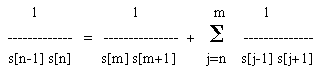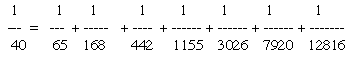# Unit Fractions and Fibonacci

Let s[j], j=0,1,2,… be a sequence of integers that satisfy the recurrence relation s[k] = s[k-1] + s[k-2] with arbitrary initial values s and s (Note that if s = 1 and s = 1, then it is the Fibonacci Sequence). Use spreadsheet software to observe that for any integers m, n with m>n we haveFor example, setting s=s=1 and n=5, m=10 gives
1/(s.s) = 1/(s.s) + 1/(s.s) +1/(s.s) +1/(s.s) +1/(s.s) +1/(s.s) +1/(s.s)
In other words,Extension: